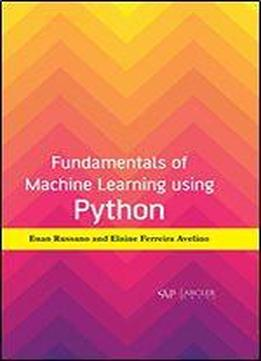# Fundamentals Of Machine Learning Using Python by Euan Russano / 2019 / English / PDF

Fundamentals of Machine Learning discusses the basics of python, use of python in computing and provides a general outlook on machine learning. This book provides an insight into concepts such as linear regression with one variable, linear algebra, and linear regression with multiple inputs. The classification with logistics regression model, regularization, neural networks, decision trees are explained in this book. The introduction to several concepts of machine learning such as component analysis, classification using k-Nearest Algorithm, k Means Clustering, computing with Tensor flow and natural language processing have been explained. This book explains the fundamental concepts of machine learning.

views: 374